Electron. J. Diff. Equ., Vol. 2015 (2015), No. 163, pp. 1-7.

### Some relations between the Caputo fractional difference operators and integer-order differences Baoguo Jia, Lynn Erbe, Allan Peterson

Abstract:
In this article, we are concerned with the relationships between the sign of Caputo fractional differences and integer nabla differences. In particular, we show that if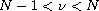,,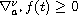, forand, thenfor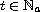. Conversely, if,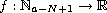, andfor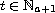, then, for each. As applications of these two results, we get that if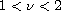,,forand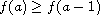, thenis an increasing function for. Conversely if,andis an increasing function for, then, for each. We also give a counterexample to show that the above assumptionin the last result is essential. These results demonstrate that, in some sense, the positivity of the-th order Caputo fractional difference has a strong connection to the monotonicity of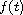.

Submitted May 30, 2015. Published June 17, 2015.
Math Subject Classifications: 39A12, 39A70.
Key Words: Caputo fractional difference; monotonicity; Taylor monomial.

Show me the PDF file (185 KB), TEX file, and other files for this article.Baoguo Jia School of Mathematics and Computer Science Sun Yat-Sen University Guangzhou 510275, China email: mcsjbg@mail.sysu.edu.cn Lynn Erbe Department of Mathematics University of Nebraska-Lincoln Lincoln, NE 68588-0130, USA email: lerbe2@math.unl.edu Allan Peterson Department of Mathematics University of Nebraska-Lincoln Lincoln, NE 68588-0130, USA email: apeterson1@math.unl.edu